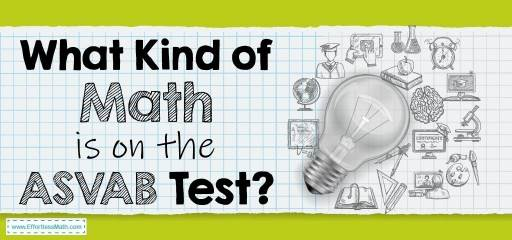# What Kind of Math Is on the ASVAB Test?The Armed Services Vocational Aptitude Battery (ASVAB) has been administered by the Department of Defense since 1968 and has been used by approximately 40 million people since then. It is a valuable talent test that measures the strengths and potential of young adults to succeed in military training.

ASVAB test scores range from 1 to 99 and all questions are multiple-choice. There are three types of ASVAB:

• The CAT-ASVAB (computer adaptive test)
• The MET-site ASVAB (paper and pencil (P&P)
• The Student ASVAB (paper and pencil (P&P)

The ASVAB test includes various sections, which include: Auto and Shop Information, General Science, Arithmetic Reasoning, Mathematics Knowledge, Word Knowledge, Paragraph Comprehension, Electronics Information, Mechanical Comprehension.

The CAT-ASVAB test is a computer and adaptive test, which means that if you answer the question correctly, the next question will be more difficult, and if you answer incorrectly, the next question will be easier. It is also not possible to go back to the previous question and correct it, while in the paper and pencil (P&P) test you can do this.

In the computer adaptive exam, there are 16 questions for each of the Arithmetic Reasoning and Mathematics Knowledge sections, but in the paper and pencil (P&P) exam there are 30 questions for the Arithmetic Reasoning section and 25 questions for the Mathematics Knowledge section. Using the calculator in the ASVAB test is not allowed.

## Arithmetic Reasoning

In the Arithmetic reasoning subtest, what matters is how good you are at solving basic arithmetic problems. In this section, you will encounter questions about word problems that you need to solve in one step or several steps. At this stage, you have the skills of addition, subtraction, multiplication, division. if necessary is more than one stage of operation, you need to know the correct order of operations. Other items that you must master in this subtest are operations with whole numbers, operations with rational numbers, ratio and proportion, interest, measurement, and percentage.

## Math Knowledge

In this section, your knowledge and understanding of the concepts of mathematics will be evaluated. You should be able to solve problems using the concepts of mathematics. Contents in this subtest include number theory, numeration, algebraic operations and equations, geometry, measurement, and probability. It is better to master the rules of algebra and geometry to get an excellent score in this area.

## More from Effortless Math for ASVAB Test …

### How do I study for the ASVAB Math?

Check out our guide on How to Prepare for the ASVAB Math Test?

### How is the ASVAB Test Scored?

We have prepared information about ASVAB Test Score here: How is the ASVAB Test Scored?

You can use our Top 10 ASVAB Math Prep Books to find the best resource for the ASVAB Math test.

## Have any questions about the ASVAB Test?

### What people say about "What Kind of Math Is on the ASVAB Test? - Effortless Math: We Help Students Learn to LOVE Mathematics"?

No one replied yet.

X
49% OFF

Limited time only!

Save Over 49%

SAVE \$34

It was \$69.99 now it is \$35.99